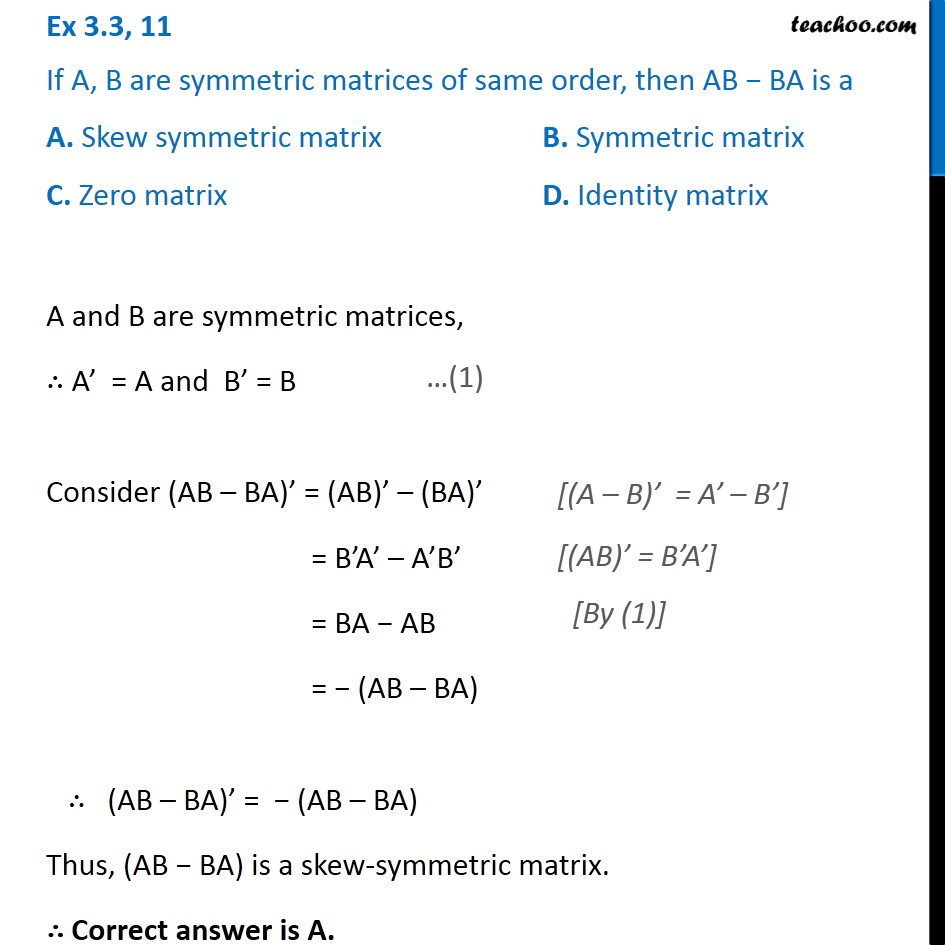1. Chapter 3 Class 12 Matrices
2. Concept wise
3. Proof using property of transpose

Transcript

Ex 3.3, 11 If A, B are symmetric matrices of same order, then AB − BA is a A. Skew symmetric matrix B. Symmetric matrix C. Zero matrix D. Identity matrix A and B are symmetric matrices, ∴ A’ = A and B’ = B Consider (AB – BA)’ = (AB)’ – (BA)’ = B’A’ – A’B’ = BA − AB = − (AB – BA) ∴ (AB – BA)’ = − (AB – BA) Thus, (AB − BA) is a skew-symmetric matrix. ∴ Correct answer is A. [(A – B)’ = A’ – B’] [(AB)’ = B’A’] [By (1)]

Proof using property of transpose

About the AuthorDavneet Singh
Davneet Singh is a graduate from Indian Institute of Technology, Kanpur. He has been teaching from the past 10 years. He provides courses for Maths and Science at Teachoo.